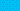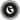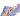PricingResources

# Current ratio vs. quick ratioWritten by

If you were ordered to pay all your creditor and supplier bills within the next 90 days, would your business be able to manage? Both the quick ratio and current ratio offer ways to assess a business’s liquidity. Here’s how to calculate each ratio along with the major differences between the pair.

What is the current ratio?

We’ll start by taking a closer look at the current ratio, which like the quick ratio is used to measure liquidity. In other words, how well is a business able to pay its current liabilities using only its current assets? There are many types of assets, but to qualify as current it must be capable of conversion into cash within a year.

Examples of current assets includes things like:

• Accounts receivable

• Cash

• Prepaid expenses

• Liquid securities

• Inventory

Like current assets, current liabilities are any debts that will be due within a year. This includes things like accounts payable, short-term debts, and accrued liabilities.

## Current ratio formula

The current ratio formula is simple:

To calculate the current ratio, you must first add up the total of all your company’s current assets and current liabilities. Divide the current asset total by the current liability total, and you’ll have your current ratio.

For example, imagine that Company ABC had a current asset total of £25,000 after adding up everything in its cash, accounts receivable, inventory, and prepaid expense accounts. It also has a current liability total of £10,000 after adding together its short-term debts and accounts payable.

The current ratio for Company ABC is 2.5, which means that it has 2.5 times its liabilities in assets and can currently meet its financial obligations Any current ratio over 2 is considered ‘good’ by most accounts.

## What is the quick ratio?

Now, let’s take a look at the quick ratio. This is also called the acid test and takes a more targeted look at how well a company can pay off its debts at this specific point in time. For assets to be included in the quick ratio, they must be convertible to cash in 90 days or less rather than a full year.

## Quick ratio formula

As with the current ratio, you use current liabilities when calculating the quick ratio. However, the quick ratio formula is a little bit different to reflect the tighter time frame involved.

Liquid securities would be any that can be converted to cash within 90 days. Current assets like inventory typically wouldn’t be included in the quick ratio formula, because they take longer than 90 days to convert to cash.

Imagine that the same Company ABC from above still holds £10,000 in current liabilities. However, when adding up its cash, accounts receivable, and liquid securities, it only has £15,000 rather than the £25,000 in current assets.

While the current ratio is 2.5, the quick ratio for Company ABC is only 1.5. This is still considered to be a good ratio. Any quick ratio over 1 means that the company holds enough in its accounts to pay off all liabilities within 90 days. If the quick ratio is under 1, this would instead indicate that the company would have difficulty paying its debts.

## Current ratio vs. quick ratio

As you can see, both the current ratio and quick ratio give useful information about a company’s asset-to-liability balance. Both ratios measure how well a business will meet its financial obligations using its existing assets. The main difference in looking at current ratio vs. quick ratio is that the quick ratio only uses the most liquid assets in its formula, while the current ratio uses all current assets.

Due to its stricter guidelines, the quick ratio is more conservative. It excludes inventory from the equation. The other major difference between the two is their target ratio. The ideal current ratio is 2:1 or greater, while the ideal quick ratio is 1:1 or greater.

## The bottom line

Both ratios are helpful for any financial analysis, but if you’re more concerned with covering short-term debt within the next 90 days you should use the quick ratio. For a longer-term view of a company’s liquidity, the current ratio provides a well-rounded view of assets vs liabilities. Ideally, you should calculate both to gain greater insight into your business’s short and long-term liquidity.

## We can help

GoCardless helps you automate payment collection, cutting down on the amount of admin your team needs to deal with when chasing invoices. Find out how GoCardless can help you with ad hoc payments or recurring payments.Contact sales

Sales

Support